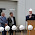Tuesday, July 17, 2018

Plot and compare two scatterplots on the same graph using R and ggplot2

If you have two sets of data located in two data frames in R, and you want to plot their correlation in a scatterplot, use the following ggplot2 code:

```ggplot(WT, aes(V1, EF, color=EF))+geom_point(shape = 16, size = 5, show.legend = FALSE, alpha = .4)
+ theme_minimal()
+ scale_color_gradient(low = "#0091ff", high = "#f0650e")
+ theme(axis.text=element_text(size=24),axis.title=element_text(size=26))
+ labs(title = "GENE WT", x="", y="Percent")
+ theme(plot.title = element_text(size = rel(2)))
+ geom_smooth(method=lm)
+ annotate(x=10,y=85,label=paste("R = ", round(cor (WT\$V1,WT\$EF),2), ", p=0.008869"),geom="text", size=8, col="darkred")```

```ggplot(KO, aes(V1, EF, color=EF))+geom_point(shape = 16, size = 5, show.legend = FALSE, alpha = .4)
+ theme_minimal()
+ scale_color_gradient(low = "#0091ff", high = "#f0650e")
+ theme(axis.text=element_text(size=24),axis.title=element_text(size=26))
+ labs(title = "GENE KO", x="Day", y="Percent")
+ theme(plot.title = element_text(size = rel(2)))
+ geom_smooth(method=lm)
+ annotate(x=10,y=85,label=paste("R = ", round(cor (KO\$V1,KO\$EF),2), ", p=1.655e-10"), geom="text", size=8, col="darkred")```

However, a better visual comparison would be if we plot these on the same graph using the code:

```ggplot() +
+ theme_minimal() +
+ theme(axis.text=element_text(size=24),axis.title=element_text(size=26))+ labs(title = "GENE KO vs. WT", x="Day", y="Percent") + theme(plot.title = element_text(size = rel(2)))+
+ geom_point(data = WT, aes(x = V1, y = EF), color = "red",shape = 16, size = 5, show.legend = FALSE, alpha = .4)+
+ geom_point(data = NAT1KO, aes(x = V1, y = EF), color = "blue",shape = 16, size = 5, show.legend = FALSE, alpha = .4)+
+ geom_smooth(data = WT, aes(x = V1, y = EF),method=lm,color="red",fill="red")+
+ geom_smooth(data = NAT1KO, aes(x = V1, y = EF),method=lm,color="blue",fill="blue")+
+ annotate(x=10,y=85,label=paste("R = ", round(cor (WT\$V1,WT\$EF),2), ", p=0.008869"),geom="text", size=8, col="red")+
+ annotate(x=7,y=47,label=paste("R = ", round(cor (NAT1KO\$V1,NAT1KO\$EF),2), ", p=1.655e-10"), geom="text", size=8, col="blue")```

1 comment:

1.Every concept with good technique have shown through this graph. Students can understand this programming part. That type of platform also available TypicalStudent.org where students can participate.